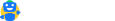accounting-and-auditing

File Tax Returns

### Expert assistance on your annual filings

Get Expert Assistance

# Process Costing – Overview, Types & Features

Updated on:

Cost Accounting refers to analyzing all costs related to a particular product in order to bring about cost effectiveness and increase profits. Based on the nature of industry, the method of cost accounting varies.   For instance: An advertising firm operates project wise and hence the cost accounting method used is Job costing. All costs related to one single project are recorded together and analyzed. Similarly, a manufacturing unit will analyze its cost based on production.

The manufacturing units can vary from one another in terms of type and number of products produced . In a unit producing spare parts,  the method of costing will be batch costing as the parts are produced in batches. Whereas in an unit producing bulk items of a standard product the method of costing will be Process Costing.

## What is process costing?

A manufacturing unit that can differentiate its processes and produces a standard product will use process costing method to determine cost of production. This is done by allocating all process cost to the total units produced.   In simple words, if an unit passes through different processes and the processes are easily distinguishable then the cost of the unit will be cost of process that it goes through.   In process costing a separate account is opened for every process and on completion of the process the cost is transferred to the next process. Illustration A paper manufacturing unit has the following processes

• Making pulp
• Beating
• Pulp to paper
• Finishing

Let us assume  the costs incurred for producing 1000 units is

 Making  pulp Rs. 10000 Beating Rs. 20000 Pulp to paper Rs. 15000 Finishing Rs. 30000 Total Costs Rs. 75000 Total units 1000 Cost per unit Rs. 75

The above illustration is a simple presentation of process costing.  However in an actual unit there will be Work in Progress at any given period end.  So the steps for process costing will be

• To determine the total production that is: Opening stock at beginning of period + Production during the period – Rejections if any +  Equivalent units of Work in process

(To convert the number of WIP units to finished product equivalent lets understand this example : Say 500 units are in WIP and they are 50% complete then the finished product equivalent will be 500*50% =250)

• To determine the total costs, both direct and indirect costs incurred for the opening stock and current production are to be considered.
• Allocation of the costs incurred for the entire inventory is done by dividing the total costs by equivalent units of production.

As we know, there will be Work in progress during the opening and closing of a period and thus an organization needs to decide on the cost flow assumptions. For computing costs under process costing the organization can use either the FIFO or weighted average cost flow assumption. Under the FIFO method it is assumed that the material that comes in first is transferred out first and hence the two methods (FIFO and weighted average) vary while treating opening inventory. The treatment of Work in progress in both the methods is as follows :

 Steps Weighted Average First in first out Total production Units transferred out+  Ending inventory WIP Beginning WIP+ Units transferred out+ ending inventory WIP Total Costs Beginning WIP costs+ costs incurred during the period Total costs incurred during the period Cost allocation Total costs /total units Total costs /total units

The above is a simple chart differentiating the two methods.  In case of WIP there will be percentage of completion that will have to be looked into and equivalent units will have to be computed. Let us understand the same with an example,  the assumption is that the percentage of completion is the same for both material and labour.

 Opening inventory 5000, 40% complete Units started during the period 12000 Closing inventory 4000,60% complete

Under the FIFO method,  the computation of total production will be as follows :

Opening inventory – 5000*60% ( since 40% has been completed) = Rs. 3000

Completed units ( Units started – closing inventory) – 12000 – 4000= Rs. 8000

Closing inventory – 4000*60%= Rs. 2400

Taking the same example let us calculate total production under the weighted average method Completed units 12000-4000= Rs. 8000 Closing WIP 4000*60%= Rs.  2400 Total production= Rs. 10400

Similarly the costs will have to be determined and assigned to the Equivalent units of production. In our day to day lives too there are many activities that can be segregated into defined processes. And the time involved can be assigned to each process. The cumulative time for all the processes will be the time of the entire activity.

## What does process costing include?

Process costing includes the following methods of costing :

#### Unit Costing

This method applies to businesses that produce a single product. In this method the total cost is divided by number of units to derive per unit cost. This is mostly used in industries dealing in- Cement manufacturing,  paper manufacturing, textiles.

#### Operating costing

This applies to service industry wherein the services are defined and distinguished. This is mostly used by  Hospitals, Railways.

#### Operation Costing

This is similar to process costing. The only difference being that instead of process the cost for various operations is considered. This is mostly used in industries dealing in Toy manufacturing Therefore process costing is widely used method of costing. It can be easily applied to manufacturing as well as service industries.Free, simple and accurate. Designed by tax expertsHave a query?
mailto@cleartax.in
Company
Individuals
Tax Experts
SMEs
Enterprises
ITR Resources
MF Resources
Tools
ClearGST
ClearServices